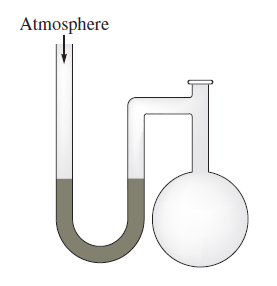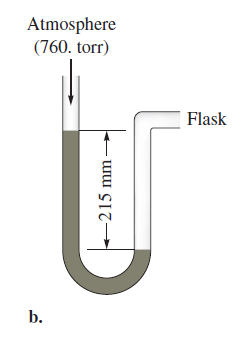# Problem: A diagram for an open-tube manometer is shown below.If the flask is open to the atmosphere, the mercury levels are equal. For each of the following situations where a gas is contained in the flask, calculate the pressure in the flask in torr, atmospheres, and pascals.If the open-tube manometer in contains a nonvolatile silicone oil (density = 1.30 g/cm3) instead of mercury (density = 13.6 g/cm3), what are the pressures in the flask in torr, atmospheres, and pascals?

⚠️Our tutors found the solution shown to be helpful for the problem you're searching for. We don't have the exact solution yet.

###### Problem Details

A diagram for an open-tube manometer is shown below.If the flask is open to the atmosphere, the mercury levels are equal. For each of the following situations where a gas is contained in the flask, calculate the pressure in the flask in torr, atmospheres, and pascals.If the open-tube manometer in contains a nonvolatile silicone oil (density = 1.30 g/cm3) instead of mercury (density = 13.6 g/cm3), what are the pressures in the flask in torr, atmospheres, and pascals?